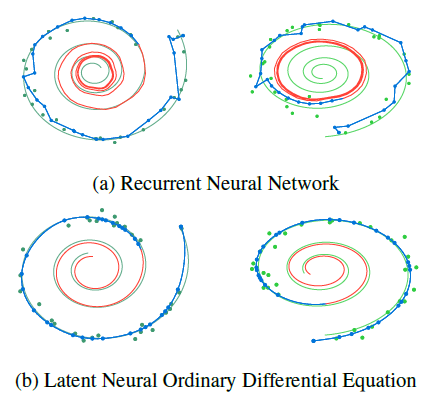# liquid_scikit_learn

Scikit learn library models to account for data and concept drift.

This python library focuses on solving data drift and concept drift in the industry to minimize retraining of the models regularly. After inspired about the capabilities of neurons in octopus tentacles, which they interact and adapt directly with the environment without their central nervous system. I designed the weights for these models in the similar way where they train on input and experience. Instead of calculating weights based on minimizing the loss function, derivatives of weights are calculated. ( Hasani Chen). This library also provides model expiration details at a feature level. This could help in finding the features that model has hard time adjusting.This library adapts concepts from Nueral ODE for scikit-learn. The models in this librabry calculate the derivatives of weights instead of weights as in standard scikit-learn librabry.

There are two training phases, the first one is a standard scikit learn model that provides predictions and weights for each feature. Typically, in standard ML models, training data is sent in batches and inferences can be done real time and in batch. In this scenario for the second training phase, input data is sent in semi batches and model adapts with changing data drift and concept drift with time. The second training phase along with changing weights it provides decay rate for each weight, contribution from data drift and concept drift and model failure parameters.

For example, suppose we train three months of data in the first training phase for the model to understand patterns with its provided inputs and outputs. In the second phase of training, we send weekly batches of inputs and outputs to make the model to adapt to changes in data and output that typically changes with customer behavior. I will make efforts to extend this library for unsupervised learning also. Currently liquid logistic regression is available with limited parameter optimization.

To use this librabry for now, git clone the librarby and give path to the librarby.

To use standard logistic regression

`from liquid_scikit_learn.liquid_logistic_regression import logistic_regression`

To use liquid logistic regression

`from liquid_scikit_learn.liquid_logistic_regression import liquid_logistic_regression`

To get model expiration details at a feature level

`from liquid_scikit_learn.liquid_logistic_regression import model_failure`

View Github